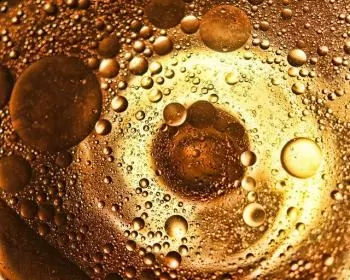# What Is Density? Formula, Definition and CharacteristicsDensity is an intensive quantity of matter in physics and chemistry that expresses how much mass of that material is present in a given volume. This physical property of matter is indicated by the Greek letter ρ.

To calculate the density, this formula is used:Where,

• m is the mass

• V is the volume

In the SI system, density is expressed in kilograms per cubic meter (kg / m3), but the oldest unit (from the cgs system) grams per cubic centimeter (g / cm3) or kilograms per cubic decimeter is also used.

To calculate the volume of an object, one can resort to the immersion bath made by Archimedes . It involves immersing the object in a container with a section that has an area that is easy to calculate and multiplying the area by the difference in height of the water surface.

The density of distilled water at a pressure of 1 atm and a temperature of 4 ° C is 1000 kilogram per cubic meter (kg / m³), ​​that is, 1 kg per liter.

## How Do Pressure and Temperature Affect Density?

The density of a substance is usually tabulated at a certain temperature and pressure because it depends on the size of these intensive quantities.

For most substances, the volume increases linearly with increasing temperature. This temperature dependence of density is expressed in the coefficient of expansion of a substance.

Water, among others, is an exception to this rule. Especially at lower temperatures, the coefficient of expansion of water shows a deviant behavior: around 4 ° C the coefficient of expansion of water has a minimum.

The mass to volume ratio of a substance generally increases linearly at higher hydrostatic pressures. This compressibility is expressed in the compression modulus. The compression modulus is closely related to the elasticity of a material, which is expressed in the elastic modulus.

## How Does Density Vary Between Gases, Liquids, and Solids?

The density of a substance differs according to the state of aggregation under standard conditions. In general, the density of a substance in the solid phase is higher than in the liquid phase.

On the other hand, that of gases is much lower than that of liquids and solids under standard conditions.

### Gases

A gas generally behaves like an ideal gas unless the pressure is very high or the temperature is well below the critical temperature of the gas. For an ideal gas, the density can be calculated using the following formula:where:

• M is the molar mass.

• P is the pressure.

• R is the gas constant.

• T is the absolute temperature.

### Liquids and Solids

Solids with an open crystalline structure have a relatively lower density than solids with a dense crystalline structure, such as metals and alloys.

As it melts, the order of the solid's crystalline structure is lost and the bonds in the crystal are broken. As a result, the average distance between atoms or molecules increases so that most substances expand during fusion. Therefore, the density of a substance usually decreases during fusion.

## Density Types

• Absolute density: expresses the relationship between the mass and the volume of a substance.

• Relative density: it is the ratio between the density of a substance and that of another reference substance and has no units. Water is usually the reference substance for solids and liquids; for gases, air.

• Apparent density: it is applied in materials that have a heterogeneous constitution. For example, porous materials contain interstices of air or other substances within them. Therefore, these materials have a lower density than if the material is compacted.

• Population density or relative population: refers to the average number of inhabitants per square kilometer of a country, region, urban or rural area in relation to a given surface unit of the territory where it is located.

Author:

Published: November 24, 2021
Last review: November 24, 2021